How Cheenta works to ensure student success?
Explore the Back-Story

# Test of Mathematics Solution Subjective 107 - Perpendiculars from CenterThis is a Test of Mathematics Solution Subjective 107 (from ISI Entrance). The book, Test of Mathematics at 10+2 Level is Published by East West Press. This problem book is indispensable for the preparation of I.S.I. B.Stat and B.Math Entrance.

Also visit: I.S.I. & C.M.I. Entrance Course of Cheenta

## Problem:

If a, b and c are the lengths of the sides of a triangle ABC and  if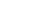and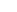are the lengths of the perpendiculars drawn from the circumcentre onto the sides BC, CA and AB respectively, then show that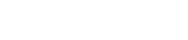.

## Solution

:
Let  O be the circum centre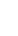= 2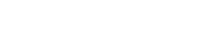Similarly,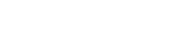&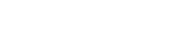Now,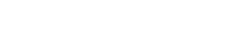=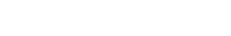=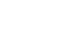=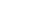Lemma
For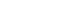&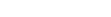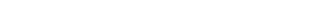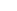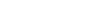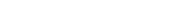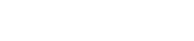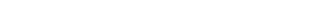(lemma proved)

So,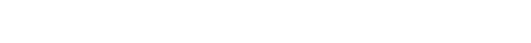=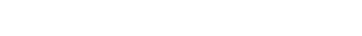[ as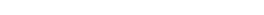]
=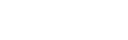=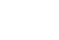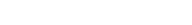( proved )This is a Test of Mathematics Solution Subjective 107 (from ISI Entrance). The book, Test of Mathematics at 10+2 Level is Published by East West Press. This problem book is indispensable for the preparation of I.S.I. B.Stat and B.Math Entrance.

Also visit: I.S.I. & C.M.I. Entrance Course of Cheenta

## Problem:

If a, b and c are the lengths of the sides of a triangle ABC and  ifandare the lengths of the perpendiculars drawn from the circumcentre onto the sides BC, CA and AB respectively, then show that.

## Solution

:
Let  O be the circum centre= 2Similarly,&Now,===Lemma
For&(lemma proved)

So,=[ as]
==( proved )

### Knowledge Partner